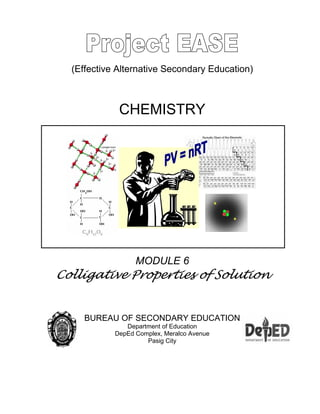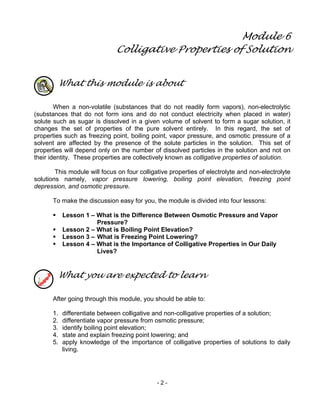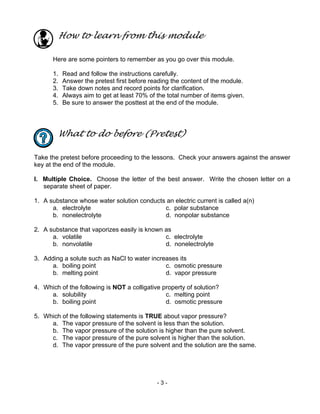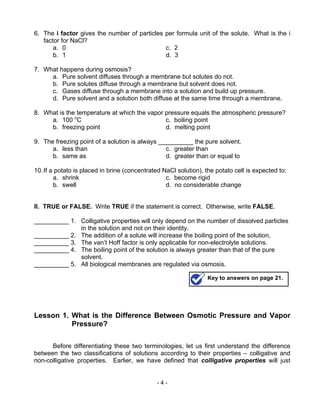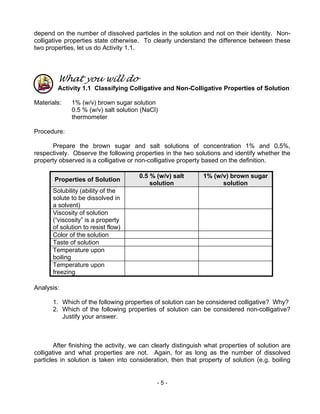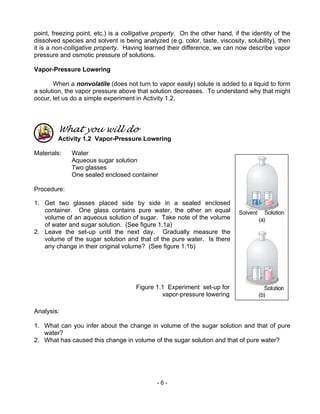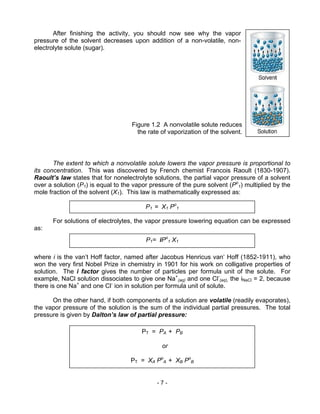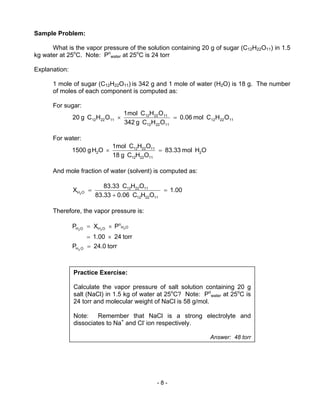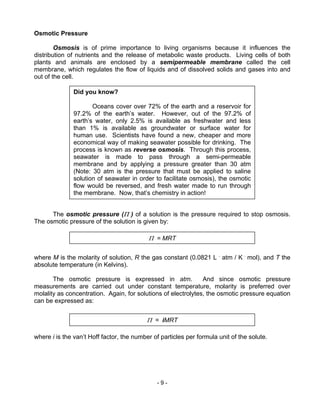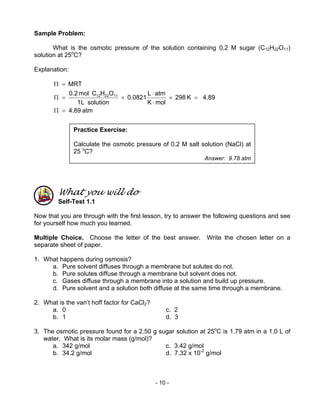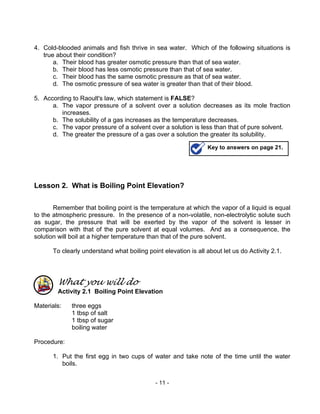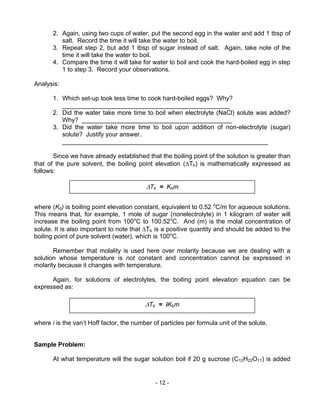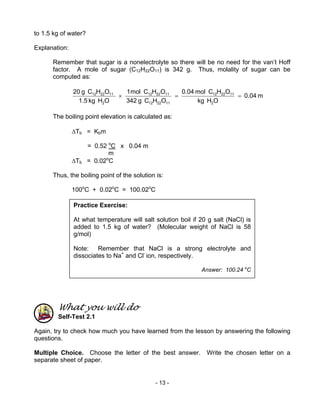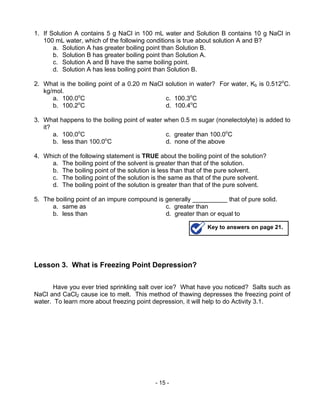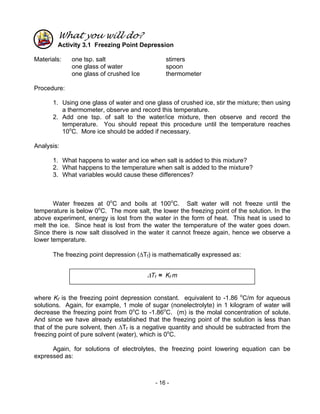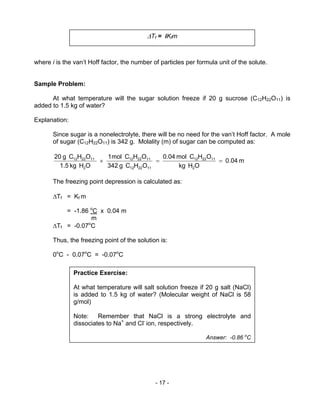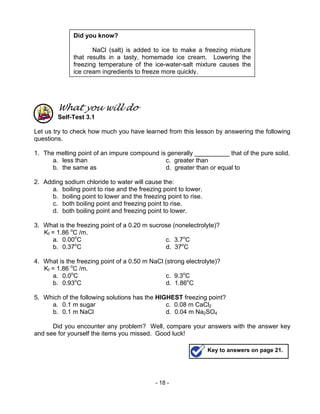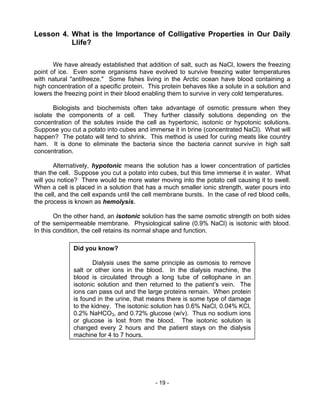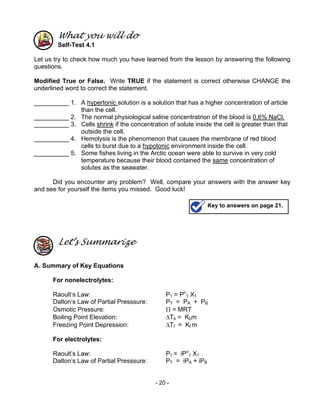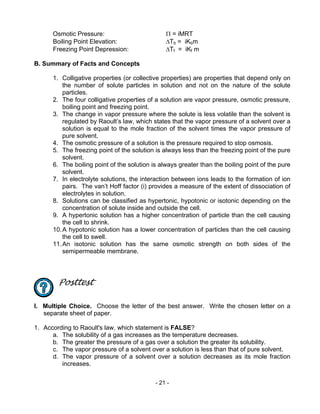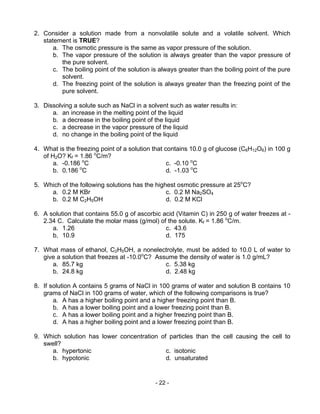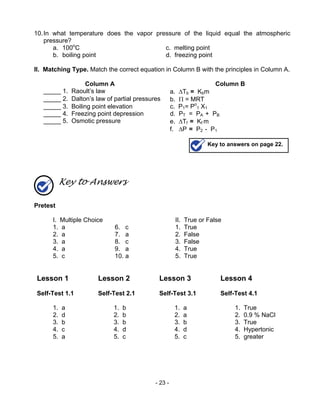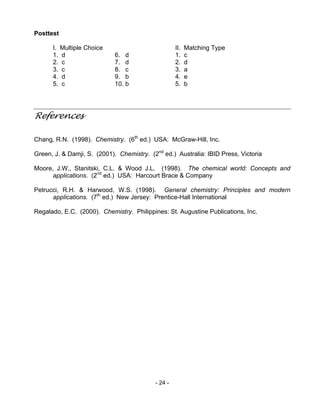Próximo SlideShare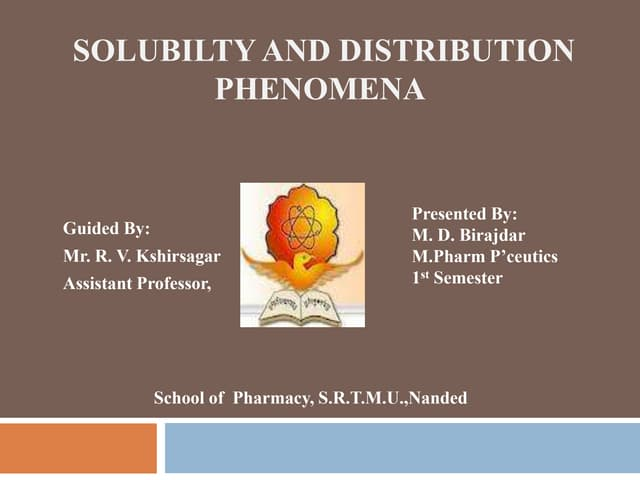Solubility & distribution phenomenon
Cargando en ... 3
1 de 24

### Chem m6 colligative properties of solution

1. (Effective Alternative Secondary Education) CHEMISTRY MODULE 6 Colligative Properties of Solution BUREAU OF SECONDARY EDUCATION Department of Education DepEd Complex, Meralco Avenue Pasig City
2. - 2 - Module 6 Colligative Properties of Solution What this module is about When a non-volatile (substances that do not readily form vapors), non-electrolytic (substances that do not form ions and do not conduct electricity when placed in water) solute such as sugar is dissolved in a given volume of solvent to form a sugar solution, it changes the set of properties of the pure solvent entirely. In this regard, the set of properties such as freezing point, boiling point, vapor pressure, and osmotic pressure of a solvent are affected by the presence of the solute particles in the solution. This set of properties will depend only on the number of dissolved particles in the solution and not on their identity. These properties are collectively known as colligative properties of solution. This module will focus on four colligative properties of electrolyte and non-electrolyte solutions namely, vapor pressure lowering, boiling point elevation, freezing point depression, and osmotic pressure. To make the discussion easy for you, the module is divided into four lessons: Lesson 1 – What is the Difference Between Osmotic Pressure and Vapor Pressure? Lesson 2 – What is Boiling Point Elevation? Lesson 3 – What is Freezing Point Lowering? Lesson 4 – What is the Importance of Colligative Properties in Our Daily Lives? What you are expected to learn After going through this module, you should be able to: 1. differentiate between colligative and non-colligative properties of a solution; 2. differentiate vapor pressure from osmotic pressure; 3. identify boiling point elevation; 4. state and explain freezing point lowering; and 5. apply knowledge of the importance of colligative properties of solutions to daily living.
4. - 4 - 6. The i factor gives the number of particles per formula unit of the solute. What is the i factor for NaCl? a. 0 c. 2 b. 1 d. 3 7. What happens during osmosis? a. Pure solvent diffuses through a membrane but solutes do not. b. Pure solutes diffuse through a membrane but solvent does not. c. Gases diffuse through a membrane into a solution and build up pressure. d. Pure solvent and a solution both diffuse at the same time through a membrane. 8. What is the temperature at which the vapor pressure equals the atmospheric pressure? a. 100 o C c. boiling point b. freezing point d. melting point 9. The freezing point of a solution is always __________ the pure solvent. a. less than c. greater than b. same as d. greater than or equal to 10.If a potato is placed in brine (concentrated NaCl solution), the potato cell is expected to: a. shrink c. become rigid b. swell d. no considerable change II. TRUE or FALSE. Write TRUE if the statement is correct. Otherwise, write FALSE. __________ 1. Colligative properties will only depend on the number of dissolved particles in the solution and not on their identity. __________ 2. The addition of a solute will increase the boiling point of the solution. __________ 3. The van’t Hoff factor is only applicable for non-electrolyte solutions. __________ 4. The boiling point of the solution is always greater than that of the pure solvent. __________ 5. All biological membranes are regulated via osmosis. Lesson 1. What is the Difference Between Osmotic Pressure and Vapor Pressure? Before differentiating these two terminologies, let us first understand the difference between the two classifications of solutions according to their properties – colligative and non-colligative properties. Earlier, we have defined that colligative properties will just Key to answers on page 21.
5. - 5 - depend on the number of dissolved particles in the solution and not on their identity. Non- colligative properties state otherwise. To clearly understand the difference between these two properties, let us do Activity 1.1. What you will do Activity 1.1 Classifying Colligative and Non-Colligative Properties of Solution Materials: 1% (w/v) brown sugar solution 0.5 % (w/v) salt solution (NaCl) thermometer Procedure: Prepare the brown sugar and salt solutions of concentration 1% and 0.5%, respectively. Observe the following properties in the two solutions and identify whether the property observed is a colligative or non-colligative property based on the definition. Properties of Solution 0.5 % (w/v) salt solution 1% (w/v) brown sugar solution Solubility (ability of the solute to be dissolved in a solvent) Viscosity of solution (“viscosity” is a property of solution to resist flow) Color of the solution Taste of solution Temperature upon boiling Temperature upon freezing Analysis: 1. Which of the following properties of solution can be considered colligative? Why? 2. Which of the following properties of solution can be considered non-colligative? Justify your answer. After finishing the activity, we can clearly distinguish what properties of solution are colligative and what properties are not. Again, for as long as the number of dissolved particles in solution is taken into consideration, then that property of solution (e.g. boiling
6. - 6 - point, freezing point, etc.) is a colligative property. On the other hand, if the identity of the dissolved species and solvent is being analyzed (e.g. color, taste, viscosity, solubility), then it is a non-colligative property. Having learned their difference, we can now describe vapor pressure and osmotic pressure of solutions. Vapor-Pressure Lowering When a nonvolatile (does not turn to vapor easily) solute is added to a liquid to form a solution, the vapor pressure above that solution decreases. To understand why that might occur, let us do a simple experiment in Activity 1.2. What you will do Activity 1.2 Vapor-Pressure Lowering Materials: Water Aqueous sugar solution Two glasses One sealed enclosed container Procedure: 1. Get two glasses placed side by side in a sealed enclosed container. One glass contains pure water, the other an equal volume of an aqueous solution of sugar. Take note of the volume of water and sugar solution. (See figure 1.1a) 2. Leave the set-up until the next day. Gradually measure the volume of the sugar solution and that of the pure water. Is there any change in their original volume? (See figure 1.1b) Analysis: 1. What can you infer about the change in volume of the sugar solution and that of pure water? 2. What has caused this change in volume of the sugar solution and that of pure water? Figure 1.1 Experiment set-up for vapor-pressure lowering
7. - 7 - After finishing the activity, you should now see why the vapor pressure of the solvent decreases upon addition of a non-volatile, non- electrolyte solute (sugar). The extent to which a nonvolatile solute lowers the vapor pressure is proportional to its concentration. This was discovered by French chemist Francois Raoult (1830-1907). Raoult’s law states that for nonelectrolyte solutions, the partial vapor pressure of a solvent over a solution (P1) is equal to the vapor pressure of the pure solvent (Po 1) multiplied by the mole fraction of the solvent (X1). This law is mathematically expressed as: For solutions of electrolytes, the vapor pressure lowering equation can be expressed as: where i is the van’t Hoff factor, named after Jacobus Henricus van’ Hoff (1852-1911), who won the very first Nobel Prize in chemistry in 1901 for his work on colligative properties of solution. The i factor gives the number of particles per formula unit of the solute. For example, NaCl solution dissociates to give one Na+ (aq) and one Cl- (aq), the iNaCl = 2, because there is one Na+ and one Cl- ion in solution per formula unit of solute. On the other hand, if both components of a solution are volatile (readily evaporates), the vapor pressure of the solution is the sum of the individual partial pressures. The total pressure is given by Dalton’s law of partial pressure: Figure 1.2 A nonvolatile solute reduces the rate of vaporization of the solvent. P1 = X1 Po 1 P1= iPo 1 X1 PT = PA + PB or PT = XA Po A + XB Po B
8. - 8 - Sample Problem: What is the vapor pressure of the solution containing 20 g of sugar (C12H22O11) in 1.5 kg water at 25o C. Note: Po water at 25o C is 24 torr Explanation: 1 mole of sugar (C12H22O11) is 342 g and 1 mole of water (H2O) is 18 g. The number of moles of each component is computed as: For sugar: OHCmol0.06 OHCg342 OHCmol1 OHCg20 112212 112212 112212 112212 =× For water: OHmol83.33 OHCg18 OHC1mol OHg1500 2 112212 112212 2 =× And mole fraction of water (solvent) is computed as: 1.00 OHC0.0683.33 OHC83.33 X 112212 112212 OH2 = + = Therefore, the vapor pressure is: torr24.0P torr241.00 PXP OH OH o OHOH 2 222 = ×= ×= Practice Exercise: Calculate the vapor pressure of salt solution containing 20 g salt (NaCl) in 1.5 kg of water at 25o C? Note: Po water at 25o C is 24 torr and molecular weight of NaCl is 58 g/mol. Note: Remember that NaCl is a strong electrolyte and dissociates to Na+ and Cl- ion respectively. Answer: 48 torr
9. - 9 - Osmotic Pressure Osmosis is of prime importance to living organisms because it influences the distribution of nutrients and the release of metabolic waste products. Living cells of both plants and animals are enclosed by a semipermeable membrane called the cell membrane, which regulates the flow of liquids and of dissolved solids and gases into and out of the cell. The osmotic pressure (Π ) of a solution is the pressure required to stop osmosis. The osmotic pressure of the solution is given by: where M is the molarity of solution, R the gas constant (0.0821 L . atm / K . mol), and T the absolute temperature (in Kelvins). The osmotic pressure is expressed in atm. And since osmotic pressure measurements are carried out under constant temperature, molarity is preferred over molality as concentration. Again, for solutions of electrolytes, the osmotic pressure equation can be expressed as: where i is the van’t Hoff factor, the number of particles per formula unit of the solute. Did you know? Oceans cover over 72% of the earth and a reservoir for 97.2% of the earth’s water. However, out of the 97.2% of earth’s water, only 2.5% is available as freshwater and less than 1% is available as groundwater or surface water for human use. Scientists have found a new, cheaper and more economical way of making seawater possible for drinking. The process is known as reverse osmosis. Through this process, seawater is made to pass through a semi-permeable membrane and by applying a pressure greater than 30 atm (Note: 30 atm is the pressure that must be applied to saline solution of seawater in order to facilitate osmosis), the osmotic flow would be reversed, and fresh water made to run through the membrane. Now, that’s chemistry in action! Π = MRT Π = iMRT
10. - 10 - Sample Problem: What is the osmotic pressure of the solution containing 0.2 M sugar (C12H22O11) solution at 25o C? Explanation: atm4.89 4.89K298 molK atmL 0.0821 solutionL1 OHCmol0.2 MRT 112212 =Π =× ⋅ ⋅ ×=Π =Π What you will do Self-Test 1.1 Now that you are through with the first lesson, try to answer the following questions and see for yourself how much you learned. Multiple Choice. Choose the letter of the best answer. Write the chosen letter on a separate sheet of paper. 1. What happens during osmosis? a. Pure solvent diffuses through a membrane but solutes do not. b. Pure solutes diffuse through a membrane but solvent does not. c. Gases diffuse through a membrane into a solution and build up pressure. d. Pure solvent and a solution both diffuse at the same time through a membrane. 2. What is the van’t hoff factor for CaCl2? a. 0 c. 2 b. 1 d. 3 3. The osmotic pressure found for a 2.50 g sugar solution at 25o C is 1.79 atm in a 1.0 L of water. What is its molar mass (g/mol)? a. 342 g/mol c. 3.42 g/mol b. 34.2 g/mol d. 7.32 x 10-2 g/mol Practice Exercise: Calculate the osmotic pressure of 0.2 M salt solution (NaCl) at 25 o C? Answer: 9.78 atm
11. - 11 - 4. Cold-blooded animals and fish thrive in sea water. Which of the following situations is true about their condition? a. Their blood has greater osmotic pressure than that of sea water. b. Their blood has less osmotic pressure than that of sea water. c. Their blood has the same osmotic pressure as that of sea water. d. The osmotic pressure of sea water is greater than that of their blood. 5. According to Raoult's law, which statement is FALSE? a. The vapor pressure of a solvent over a solution decreases as its mole fraction increases. b. The solubility of a gas increases as the temperature decreases. c. The vapor pressure of a solvent over a solution is less than that of pure solvent. d. The greater the pressure of a gas over a solution the greater its solubility. Lesson 2. What is Boiling Point Elevation? Remember that boiling point is the temperature at which the vapor of a liquid is equal to the atmospheric pressure. In the presence of a non-volatile, non-electrolytic solute such as sugar, the pressure that will be exerted by the vapor of the solvent is lesser in comparison with that of the pure solvent at equal volumes. And as a consequence, the solution will boil at a higher temperature than that of the pure solvent. To clearly understand what boiling point elevation is all about let us do Activity 2.1. What you will do Activity 2.1 Boiling Point Elevation Materials: three eggs 1 tbsp of salt 1 tbsp of sugar boiling water Procedure: 1. Put the first egg in two cups of water and take note of the time until the water boils. Key to answers on page 21.